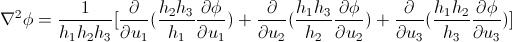## Pages

Just thought of reviewing the gradient, divergence and the curl in the curvilinear system. I must be clear that the general form I post here is the special case of the curvilinear system. That is to say that, it is valid only if the given set of the axes are orthogonal to each other. (for e.g Polar system, Spherical system, Cylindrical system or any other the orthogonal system). The reason I note down this special sytem is that it turns out that these orthogonal sets seem to be very important in practice.
Suppose $\phi$ is a scalar function and $\vec{A}=A_{1}e_{1}+A_{2}e_{2}+A_{3}e_{3}$ is a vecto function of orthogonal curvilinear co-ordinates $u_{1},u_{2},u_{3}$. Then the following laws holds,

###A simple tutorial notes on this subject matter can be found here. Some examples have been worked out too.

Some notes on the symbols used,

$h_{1},h_{2},h_{3}$ are the scale factors. $u_{1},u_{2},u_{3}$ are the coordinate axes. $e_{1},e_{2},e_{3}$ are the directional vector in their respective orthogonal coordinate axes.